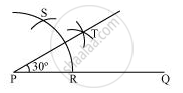# Construct the angles of the following measurement:- 30° - Mathematics

Construct the angles of the following measurement:- 30°

#### Solution

The below given steps will be followed to construct an angle of 30°.

Step I: Draw the given ray PQ. Taking P as centre and with some radius, draw an arc of a circle which intersects PQ at R.

Step II: Taking R as centre and with the same radius as before, draw an arc intersecting the previously drawn arc at point S.

Step III: Taking R and S as centre and with radius more than 1/2RS, draw arcs to intersect each other at T. Join PT which is the required ray making 30° with the given ray PQ.Concept: Basic Constructions
Is there an error in this question or solution?
Chapter 11: Constructions - Exercise 11.1 [Page 191]

#### APPEARS IN

NCERT Class 9 Maths
Chapter 11 Constructions
Exercise 11.1 | Q 3.1 | Page 191

Share